## Positional Systems and Bases

### Learning Outcomes

• Become familiar with the history of positional number systems
• Identify bases that have been used in number systems historically
• Convert numbers between bases
• Use two different methods for converting numbers between bases

More important than the form of the number symbols is the development of the place value system. Although it is in slight dispute, the earliest known document in which the Indian system displays a positional system dates back to 346 CE. However, some evidence suggests that they may have actually developed a positional system as far back as the first century CE.

In this lesson we will explore positional systems an their historical development.  We will also discuss some of the positional systems that have been used throughout history and the bases used for those systems.  Finally, we will learn how to convert numbers between bases and systems.

## The Positional System and Base 10

The Indians were not the first to use a positional system. The Babylonians (as we will see in Chapter 3) used a positional system with 60 as their base. However, there is not much evidence that the Babylonian system had much impact on later numeral systems, except with the Greeks. Also, the Chinese had a base-10 system, probably derived from the use of a counting board. Some believe that the positional system used in India was derived from the Chinese system.

Wherever it may have originated, it appears that around 600 CE, the Indians abandoned the use of symbols for numbers higher than nine and began to use our familiar system where the position of the symbol determines its overall value. Numerous documents from the seventh century demonstrate the use of this positional system.

Interestingly, the earliest dated inscriptions using the system with a symbol for zero come from Cambodia. In 683, the 605th year of the Saka era is written with three digits and a dot in the middle. The 608th year uses three digits with a modern 0 in the middle. The dot as a symbol for zero also appears in a Chinese work (Chiu-chih li). The author of this document gives a strikingly clear description of how the Indian system works:

Using the [Indian] numerals, multiplication and division are carried out. Each numeral is written in one stroke. When a number is counted to ten, it is advanced into the higher place. In each vacant place a dot is always put. Thus the numeral is always denoted in each place. Accordingly there can be no error in determining the place. With the numerals, calculations is easy.

### Transmission to Europe

It is not completely known how the system got transmitted to Europe. Traders and travelers of the Mediterranean coast may have carried it there. It is found in a tenth-century Spanish manuscript and may have been introduced to Spain by the Arabs, who invaded the region in 711 CE and were there until 1492.

In many societies, a division formed between those who used numbers and calculation for practical, every day business and those who used them for ritualistic purposes or for state business. The former might often use older systems while the latter were inclined to use the newer, more elite written numbers. Competition between the two groups arose and continued for quite some time.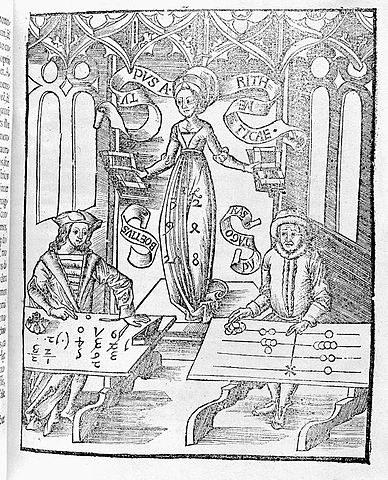Figure 15.

In a fourteenth century manuscript of Boethius’ The Consolations of Philosophy, there appears a well-known drawing of two mathematicians. One is a merchant and is using an abacus (the “abacist”). The other is a Pythagorean philosopher (the “algorist”) using his “sacred” numbers. They are in a competition that is being judged by the goddess of number. By 1500 CE, however, the newer symbols and system had won out and has persevered until today. The Seattle Times recently reported that the Hindu-Arabic numeral system has been included in the book The Greatest Inventions of the Past 2000 Years.

One question to answer is why the Indians would develop such a positional notation. Unfortunately, an answer to that question is not currently known. Some suggest that the system has its origins with the Chinese counting boards. These boards were portable and it is thought that Chinese travelers who passed through India took their boards with them and ignited an idea in Indian mathematics. Others, such as G. G. Joseph propose that it is the Indian fascination with very large numbers that drove them to develop a system whereby these kinds of big numbers could easily be written down. In this theory, the system developed entirely within the Indian mathematical framework without considerable influence from other civilizations.

## The Development and Use of Different Number Bases

### Introduction and Basics

During the previous discussions, we have been referring to positional base systems. In this section of the chapter, we will explore exactly what a base system is and what it means if a system is “positional.” We will do so by first looking at our own familiar, base-ten system and then deepen our exploration by looking at other possible base systems. In the next part of this section, we will journey back to Mayan civilization and look at their unique base system, which is based on the number 20 rather than the number 10.

A base system is a structure within which we count. The easiest way to describe a base system is to think about our own base-ten system. The base-ten system, which we call the “decimal” system, requires a total of ten different symbols/digits to write any number. They are, of course, 0, 1, 2, . . . , 9.

The decimal system is also an example of a positional base system, which simply means that the position of a digit gives its place value. Not all civilizations had a positional system even though they did have a base with which they worked.

In our base-ten system, a number like 5,783,216 has meaning to us because we are familiar with the system and its places. As we know, there are six ones, since there is a 6 in the ones place. Likewise, there are seven “hundred thousands,” since the 7 resides in that place. Each digit has a value that is explicitly determined by its position within the number. We make a distinction between digit, which is just a symbol such as 5, and a number, which is made up of one or more digits. We can take this number and assign each of its digits a value. One way to do this is with a table, which follows:

 5,000,000 = 5 × 1,000,000 = 5 × 106 Five million +700,000 = 7 × 100,000 = 7 × 105 Seven hundred thousand +80,000 = 8 × 10,000 = 8 × 104 Eighty thousand +3,000 = 3 × 1000 = 3 × 103 Three thousand +200 = 2 × 100 = 2 × 102 Two hundred +10 = 1 × 10 = 1 × 101 Ten +6 = 6 × 1 = 6 × 100 Six 5,783,216 Five million, seven hundred eighty-three thousand, two hundred sixteen

From the third column in the table we can see that each place is simply a multiple of ten. Of course, this makes sense given that our base is ten. The digits that are multiplying each place simply tell us how many of that place we have. We are restricted to having at most 9 in any one place before we have to “carry” over to the next place. We cannot, for example, have 11 in the hundreds place. Instead, we would carry 1 to the thousands place and retain 1 in the hundreds place. This comes as no surprise to us since we readily see that 11 hundreds is the same as one thousand, one hundred. Carrying is a pretty typical occurrence in a base system.

However, base-ten is not the only option we have. Practically any positive integer greater than or equal to 2 can be used as a base for a number system. Such systems can work just like the decimal system except the number of symbols will be different and each position will depend on the base itself.

### Other Bases

For example, let’s suppose we adopt a base-five system. The only modern digits we would need for this system are 0,1,2,3 and 4. What are the place values in such a system? To answer that, we start with the ones place, as most base systems do. However, if we were to count in this system, we could only get to four (4) before we had to jump up to the next place. Our base is 5, after all! What is that next place that we would jump to? It would not be tens, since we are no longer in base-ten. We’re in a different numerical world. As the base-ten system progresses from 100 to 101, so the base-five system moves from 50 to 51 = 5. Thus, we move from the ones to the fives.

After the fives, we would move to the 52 place, or the twenty fives. Note that in base-ten, we would have gone from the tens to the hundreds, which is, of course, 102.

Let’s take an example and build a table. Consider the number 30412 in base five. We will write this as 304125, where the subscript 5 is not part of the number but indicates the base we’re using. First off, note that this is NOT the number “thirty thousand, four hundred twelve.” We must be careful not to impose the base-ten system on this number. Here’s what our table might look like. We will use it to convert this number to our more familiar base-ten system.

 Base 5 This column coverts to base-ten In Base-Ten 3 × 54 = 3 × 625 = 1875 + 0 × 53 = 0 × 125 = 0 + 4 × 52 = 4 × 25 = 100 + 1 × 51 = 1 × 5 = 5 + 2 × 50 = 2 × 1 = 2 Total 1982

As you can see, the number 304125 is equivalent to 1,982 in base-ten. We will say 304125 = 198210. All of this may seem strange to you, but that’s only because you are so used to the only system that you’ve ever seen.

### Example

Convert 62347 to a base 10 number.

### Try It

Convert 410657 to a base 10 number.

Watch this video to see more examples of converting numbers in bases other than 10 into a base 10 number.

## Another Method For Converting From Base 10 to Other Bases

As you read the solution to this last example and attempted the “Try It” problems, you may have had to repeatedly stop and think about what was going on. The fact that you are probably struggling to follow the explanation and reproduce the process yourself is mostly due to the fact that the non-decimal systems are so unfamiliar to you. In fact, the only system that you are probably comfortable with is the decimal system.

As budding mathematicians, you should always be asking questions like “How could I simplify this process?” In general, that is one of the main things that mathematicians do: they look for ways to take complicated situations and make them easier or more familiar. In this section we will attempt to do that.

To do so, we will start by looking at our own decimal system. What we do may seem obvious and maybe even intuitive but that’s the point. We want to find a process that we readily recognize works and makes sense to us in a familiar system and then use it to extend our results to a different, unfamiliar system.

Let’s start with the decimal number, 486310. We will convert this number to base 10. Yeah, I know it’s already in base 10, but if you carefully follow what we’re doing, you’ll see it makes things work out very nicely with other bases later on. We first note that the highest power of 10 that will divide into 4863 at least once is 103 = 1000. In general, this is the first step in our new process; we find the highest power that a given base that will divide at least once into our given number.

We now divide 1000 into 4863:

4863 ÷ 1000 = 4.863

This says that there are four thousands in 4863 (obviously). However, it also says that there are 0.863 thousands in 4863. This fractional part is our remainder and will be converted to lower powers of our base (10). If we take that decimal and multiply by 10 (since that’s the base we’re in) we get the following:

0.863 × 10 = 8.63

Why multiply by 10 at this point? We need to recognize here that 0.863 thousands is the same as 8.63 hundreds. Think about that until it sinks in.

(0.863)(1000) = 863
(8.63)(100) = 863

These two statements are equivalent. So, what we are really doing here by multiplying by 10 is rephrasing or converting from one place (thousands) to the next place down (hundreds).

0.863 × 10 ⇒ 8.63
(Parts of Thousands) × 10 ⇒ Hundreds

What we have now is 8 hundreds and a remainder of 0.63 hundreds, which is the same as 6.3 tens. We can do this again with the 0.63 that remains after this first step.

0.63 × 10 ⇒ 6.3
Hundreds × 10 ⇒ Tens

So we have six tens and 0.3 tens, which is the same as 3 ones, our last place value.

Now here’s the punch line. Let’s put all of the together in one place: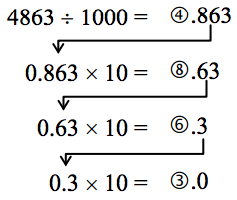Converting from Base 10 to Base b: Another methodNote that in each step, the remainder is carried down to the next step and multiplied by 10, the base. Also, at each step, the whole number part, which is circled, gives the digit that belongs in that particular place. What is amazing is that this works for any base! So, to convert from a base 10 number to some other base, b, we have the following steps we can follow:

### Converting from Base 10 to Base b: Another method

1. Find the highest power of the base b that will divide into the given number at least once and then divide.
2. Keep the whole number part, and multiply the fractional part by the base b.
3. Repeat step two, keeping the whole number part (including 0), carrying the fractional part to the next step until only a whole number result is obtained.
4. Collect all your whole number parts to get your number in base b notation.

We will illustrate this procedure with some examples.

### Example

Convert the base 10 number, 34810, to base 5.

We can compare our result with what we saw earlier, or simply check with our calculator, and find that these two numbers really are equivalent to each other.

### Example

Convert the base 10 number, 300710, to base 5.

This last example shows the importance of using a calculator in certain situations and taking care to avoid clearing the calculator’s memory or display until you get to the very end of the process.

### Example

Convert the base 10 number, 6320110, to base 7.

If we round, even to two decimal places in each step, clearing our calculator out at each step along the way, we will get a series of numbers that do not terminate, but begin repeating themselves endlessly. (Try it!) We end up with something that doesn’t make any sense, at least not in this context. So be careful to use your calculator cautiously on these conversion problems.

Also, remember that if your first division is by 75, then you expect to have 6 digits in the final answer, corresponding to the places for 75, 74, and so on down to 70. If you find yourself with more than 6 digits due to rounding errors, you know something went wrong.

### Try It

Convert the base 10 number, 935210, to base 5.

Convert the base 10 number, 1500, to base 3.

Be careful not to clear your calculator on this one. Also, if you’re not careful in each step, you may not get all of the digits you’re looking for, so move slowly and with caution.

The following video shows how to use a calculator to convert numbers in base 10 into other bases.

## The Mayan Numeral System

### Background

As you might imagine, the development of a base system is an important step in making the counting process more efficient. Our own base-ten system probably arose from the fact that we have 10 fingers (including thumbs) on two hands. This is a natural development. However, other civilizations have had a variety of bases other than ten. For example, the Natives of Queensland used a base-two system, counting as follows: “one, two, two and one, two two’s, much.” Some Modern South American Tribes have a base-five system counting in this way: “one, two, three, four, hand, hand and one, hand and two,” and so on. The Babylonians used a base-sixty (sexigesimal) system. In this chapter, we wrap up with a specific example of a civilization that actually used a base system other than 10.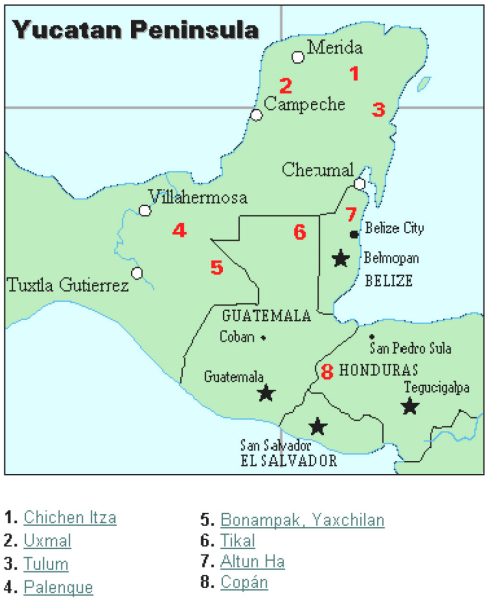The Mayan civilization is generally dated from 1500 BCE to 1700 CE. The Yucatan Peninsula (see figure 16) in Mexico was the scene for the development of one of the most advanced civilizations of the ancient world. The Mayans had a sophisticated ritual system that was overseen by a priestly class. This class of priests developed a philosophy with time as divine and eternal. The calendar, and calculations related to it, were thus very important to the ritual life of the priestly class, and hence the Mayan people. In fact, much of what we know about this culture comes from their calendar records and astronomy data. Another important source of information on the Mayans is the writings of Father Diego de Landa, who went to Mexico as a missionary in 1549.There were two numeral systems developed by the Mayans—one for the common people and one for the priests. Not only did these two systems use different symbols, they also used different base systems. For the priests, the number system was governed by ritual. The days of the year were thought to be gods, so the formal symbols for the days were decorated heads, like the sample to the left Since the basic calendar was based on 360 days, the priestly numeral system used a mixed base system employing multiples of 20 and 360. This makes for a confusing system, the details of which we will skip.

 Powers Base-Ten Value Place Name 207 12,800,000,000 Hablat 206 64,000,000 Alau 205 3,200,000 Kinchil 204 160,000 Cabal 203 8,000 Pic 202 400 Bak 201 20 Kal 200 1 Hun

### The Mayan Number System

Instead, we will focus on the numeration system of the “common” people, which used a more consistent base system. As we stated earlier, the Mayans used a base-20 system, called the “vigesimal” system. Like our system, it is positional, meaning that the position of a numeric symbol indicates its place value. In the following table you can see the place value in its vertical format.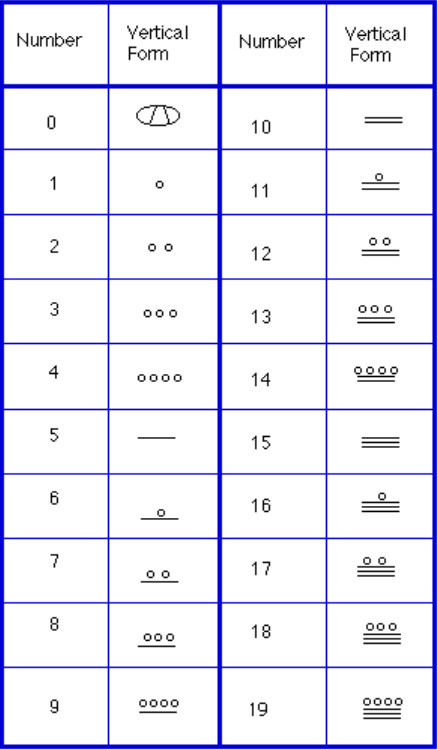In order to write numbers down, there were only three symbols needed in this system. A horizontal bar represented the quantity 5, a dot represented the quantity 1, and a special symbol (thought to be a shell) represented zero. The Mayan system may have been the first to make use of zero as a placeholder/number. The first 20 numbers are shown in the table to the right.

Unlike our system, where the ones place starts on the right and then moves to the left, the Mayan systems places the ones on the bottom of a vertical orientation and moves up as the place value increases.

When numbers are written in vertical form, there should never be more than four dots in a single place. When writing Mayan numbers, every group of five dots becomes one bar. Also, there should never be more than three bars in a single place…four bars would be converted to one dot in the next place up. It’s the same as 10 getting converted to a 1 in the next place up when we carry during addition.

### Example

What is the value of this number, which is shown in vertical form?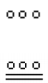### Example

What is the value of the following Mayan number?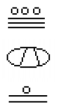### Try It

Convert the Mayan number below to base 10.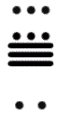### Example

Convert the base 10 number 357510 to Mayan numerals.

### Writing numbers with bases bigger than 10

When the base of a number is larger than 10, separate each “digit” with a comma to make the separation of digits clear.

For example, in base 20, to write the number corresponding to 17 × 202 + 6 × 201 + 13 × 200, we’d write 17,6,1320.

### Try It

Convert the base 10 number 1055310 to Mayan numerals.

Convert the base 10 number 561710 to Mayan numerals.

In the following video we present more examples of how to write numbers using Mayan numerals as well as converting numerals written in Mayan for into base 10 form.

The next video shows more examples of converting base 10 numbers into Mayan numerals.

When adding Mayan numbers together, we’ll adopt a scheme that the Mayans probably did not use but which will make life a little easier for us.

### Example

Add, in Mayan, the numbers 37 and 29: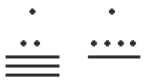### Try It

Try adding 174 and 78 in Mayan by first converting to Mayan numbers and then working entirely within that system. Do not add in base-ten (decimal) until the very end when you check your work.